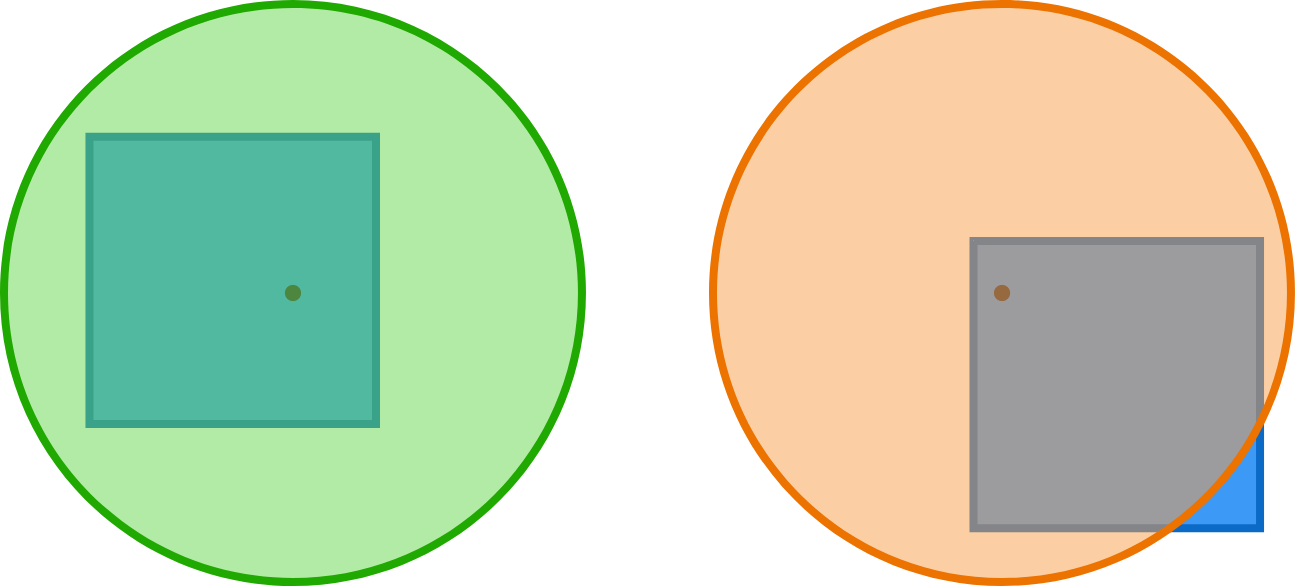# Unit Circle on Unit Square

Geometry Level 2

Select a random point from the interior of a unit square (uniformly across the area). Let $p$ be the probability that a unit circle centered at that point will completely cover the square.

To three decimal places, what is $p?$On the left, the unit circle completely covers the unit square. On the right, the unit circle does not completely cover the unit square.

×